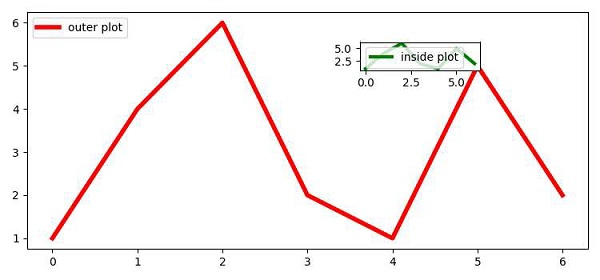# Embedding small plots inside subplots in Matplotlib

To embed small plots inside subplots, we can take the following Steps −

• Using subplots() method, create a figure and a set of subplots (fig, ax1).

• On ax1, plot a line with color red, line width=4, label=”outer plot”.

• Using add_axes(), add an axis, i.e., ax2 with l, b, h and w values.

• Plot the same points (Step 2) usins the plot() method, with color green, line width=3, label=”inside plot”.

• Set the legend on both the plots using the legend() method.

• To display the figure, use the show() method.

## Example

from matplotlib import pyplot as plt
plt.rcParams["figure.figsize"] = [7.50, 3.50]
plt.rcParams["figure.autolayout"] = True
fig, ax1 = plt.subplots()
ax1.plot([1, 4, 6, 2, 1, 5, 2], c="red", lw=4, label="outer plot")
l, b, h, w = .6, .75, .1, .2
ax2 = fig.add_axes([l, b, w, h])
ax2.plot([1, 4, 6, 2, 1, 5, 2], color='green', lw=3, label="inside plot")
ax1.legend(loc='upper left')
ax2.legend(loc='upper left')
plt.show()

## Output